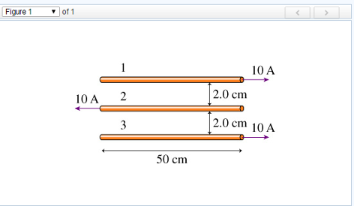# Problem: Part AWhat is the force magnitude on the first wire in the figure? (Figure 1)Part BWhat is the force magnitude on the second wire in the figure?Part CWhat is the force magnitude on the third wire in the figure?

###### FREE Expert Solution

The force magnitude:

$\overline{){\mathbf{F}}{\mathbf{=}}{\mathbit{B}}{\mathbit{i}}{\mathbit{L}}{\mathbf{=}}{\mathbf{\left(}}\frac{{\mathbf{\mu }}_{\mathbf{0}}\mathbf{i}}{\mathbf{2}\mathbf{\pi }\mathbf{r}}{\mathbf{\right)}}{\mathbit{i}}{\mathbit{L}}}$

The force is attractive when the currents flow in the same direction.

The force is repulsive when the currents flow in opposite directions.

Part A

${{\mathbf{F}}}_{{\mathbf{12}}}{\mathbf{=}}{\mathbf{\left(}}\frac{{\mathbf{\mu }}_{\mathbf{0}}{\mathbf{i}}_{\mathbf{2}}}{\mathbf{2}{\mathbf{\pi r}}_{\mathbf{12}}}{\mathbf{\right)}}{{\mathbit{i}}}_{{\mathbf{1}}}{{\mathbit{L}}}_{{\mathbf{1}}}{\mathbf{=}}\frac{\mathbf{\left(}\mathbf{4}\mathbf{\pi }\mathbf{×}{\mathbf{10}}^{\mathbf{-}\mathbf{7}}\mathbf{\right)}\mathbf{\left(}\mathbf{10}\mathbf{\right)}}{\mathbf{2}\mathbf{\pi }\mathbf{\left(}\mathbf{2}\mathbf{×}{\mathbf{10}}^{\mathbf{-}\mathbf{2}}\mathbf{\right)}}{\mathbf{×}}{\mathbf{\left(}}{\mathbf{10}}{\mathbf{\right)}}{\mathbf{\left(}}{\mathbf{50}}{\mathbf{×}}{{\mathbf{10}}}^{\mathbf{-}\mathbf{2}}{\mathbf{\right)}}$

F12 = 5.0 × 10-4 N

95% (29 ratings)###### Problem DetailsPart A

What is the force magnitude on the first wire in the figure? (Figure 1)

Part B

What is the force magnitude on the second wire in the figure?

Part C

What is the force magnitude on the third wire in the figure?